1. Home
2. Blog

# Calculation of Percentage ionization of weak electrolytes

by egpat       19 Mar 2020

Sometimes we need to know the status of ionization of an organic compound in order to assess few of the physicochemical properties of the compound. For example, if the compound is more ionized at a particular pH, it will have more water solubility. On the other hand, if we concentrate on the permeability of the compound across a physiological membrane, then unionized form of the compound is more important as it will be more lipophilic and can cross the lipophilic membrane.

So, calculation of percent ionization is an essential task we come across many times while we deal with pharmacokinetics of drugs. Here let’s see how we can calculate it along with few examples.

## Henderson-Hasselbalch equation

Let’s take a weak electrolyte such as HA which acts like weak acid. When it dissolved in water, it is not completely ionised but achieves equilibrium so that it can exist both in ionized as well as unionised forms.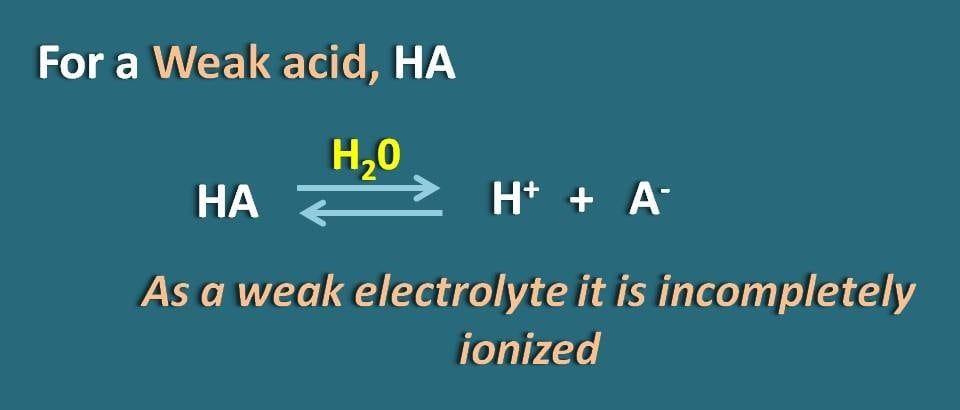By applying Hendersen-Hasselbach equation for the weak acid, we can relate the pH of the solution with the pKa of the drug and its ionization.

So, pH=pKa + log(A-/HA)

Where A- is the ionized form the weak acid whereas HA is the unionized form.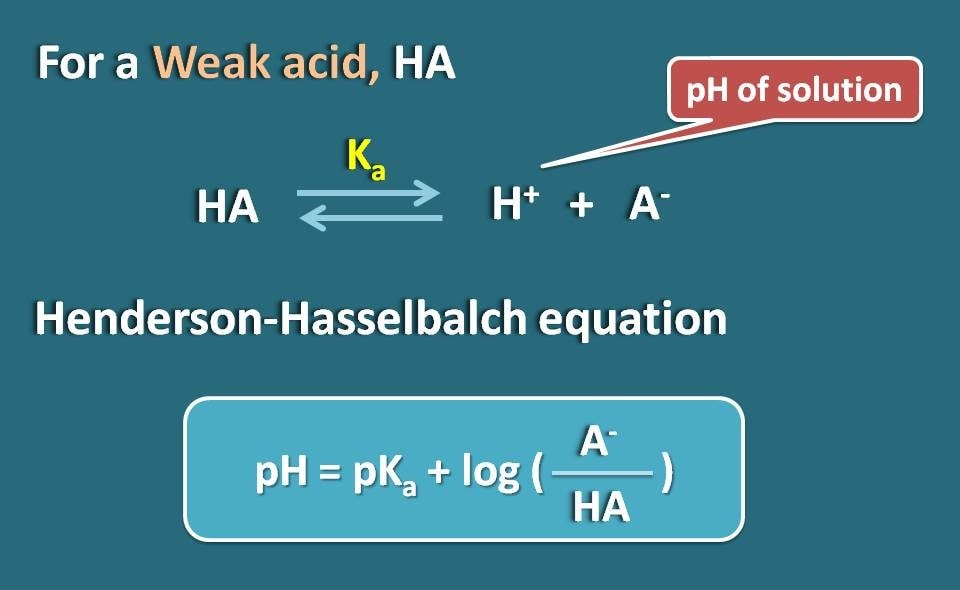Now let’s rearrange this equation so that we will get the ratio of ionized to unionized forms of the weak acid.

log(A-/HA)=pH - pKa

Taking antilogarithm,

A-/HA=10(pH – pKa)

Now, we know the ratio of ionized form to unionized form. From this we can calculate the percent ionization.

% ionized=[Ionized/(Ionized +Unionized)] * 100

Subsituting in this equation,

% ionized=[10(pH – pKa)/ (10(pH – pKa)+1)]* 100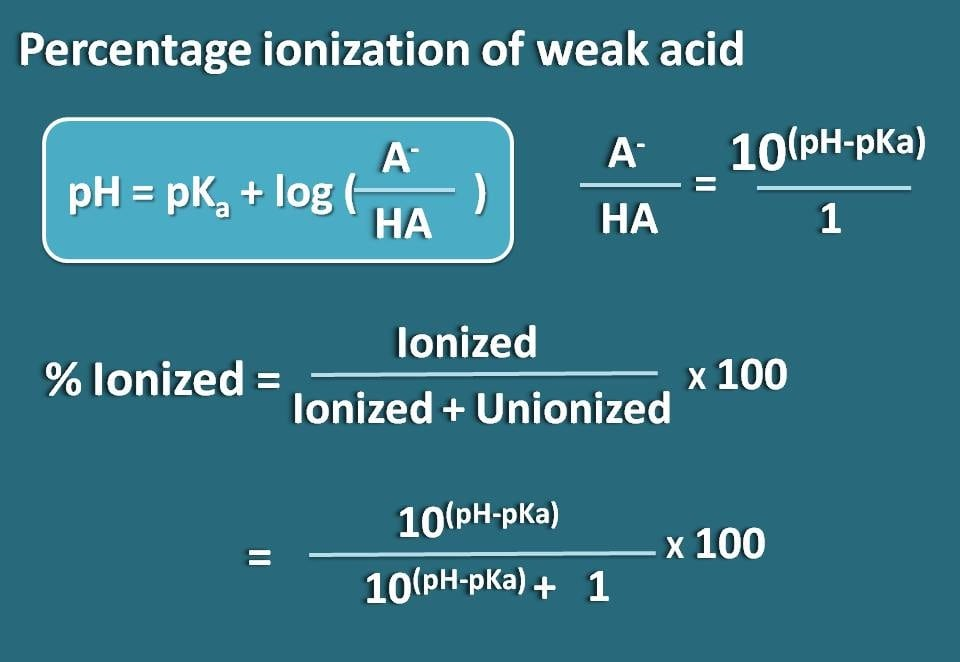Similarly we can obtain the formula for a weak base like B whose conjugate acid is BH+. Now we can write chemical equilibrium for the conjugate acid as below.

Now writing Hendersen-Hasselbalch equation for the conjugate acid

pH=pKa + log (B/BH+)

Now the ratio of unionized to ionized forms of the base can be written as

B/BH+=10(pH – pKa)

Here the portion of unionized form is 10(pH – pKa) whereas ionized form is 1. So the percentage ionization will be

% ionized=[1/ (1+10(pH – pKa))]* 100Alternatively, we can also rearrange the above equation by reversing the term pH-pKa into pKa-pH where we get the formula as

% ionized=[10(pKa  - pH)/ (10(pka – pH) + 1)]* 100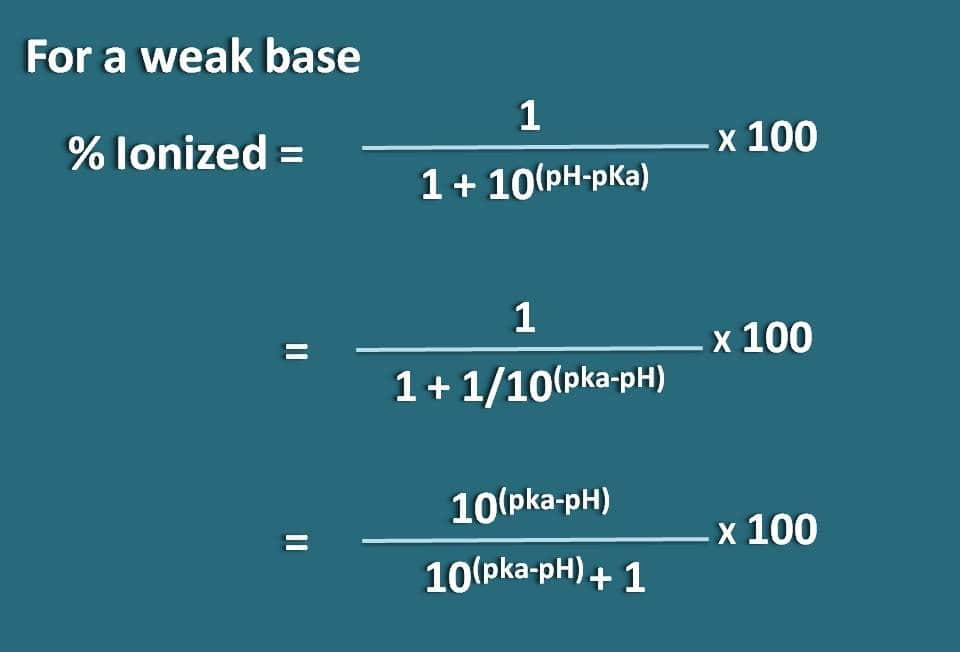Now let’s go with few of the practical examples and let’s see how can we solve them.

## Working Example 1

Calculate percentage ionized of a weakly acidic drug at a pH of 4.6 with pKa value as 8.6.

Solution:

First of let’s list out the data given.

pH=4.6 and pKa=8.6

Since it is a weakly acidic drug, let’s apply the following formula.Substituting in the above equation,

% ionized=[10(4.6 – 8.6)/ (10(4.6 – 8.6)+1)]* 100

=1/1.01=0.99 %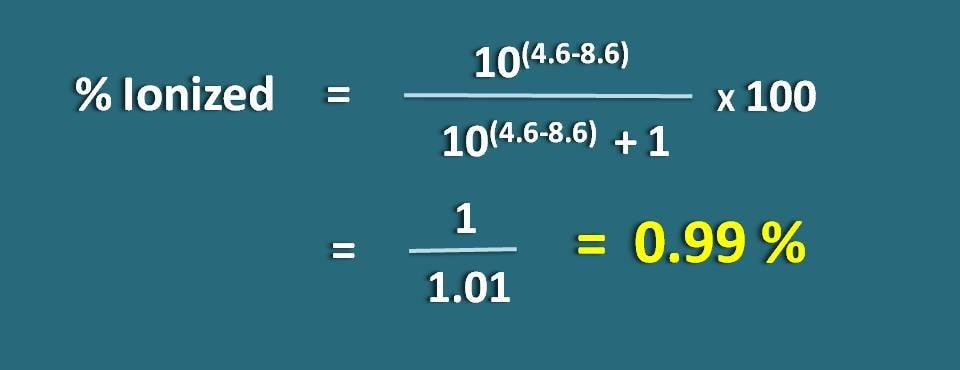Let’s go with another example.

## Working Example 2

Calculate the fraction of drug in the ionized form at pH 7.7 of a tissue for a basic drug with pKa 8.7.

Solution:

First of let’s list out the data given.

pH=7.7 and pKa=8.7

Here it is a weak base, so we have to apply the following formula.Substituting in the above equation,

% ionized=[1/ ( 1+ 10(7.7 – 8.7))]* 100

=100/1.1=90.9 %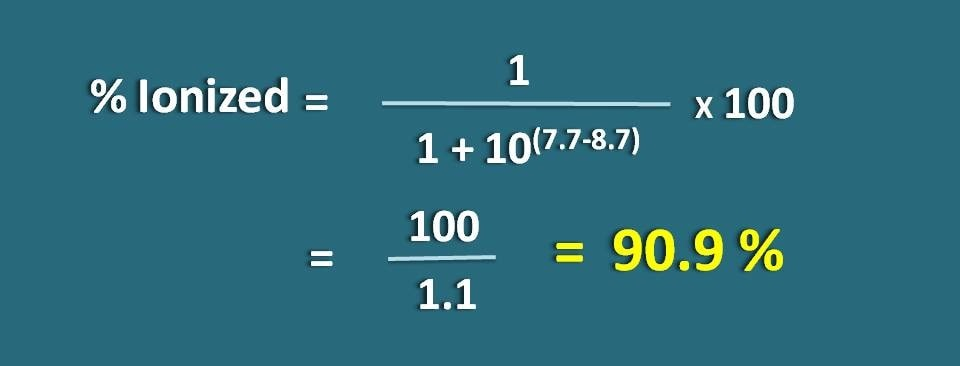Finally we conclude with a different example.

## Working Example 3

Calculate the ratio of protonated to unprotonated forms of morphine at pH 5. pKa  of morphine is 7.

Solution:

Again the date given is pH=5 and pKa=7.

Morphine is an opioid analgesic that has tertiary amine in the ring hence acts as weak base. But here the ratio of protonated to unprotonated forms of the drug was asked. Protonated form is nothing but the ionized form while unprotonated form is the unionized form. For a weak base the ratio will be 10(pH – pKa) : 1 .and let’s substitute the values.

Protonated/ unprotonated=10(5 – 7)/1

=100 : 1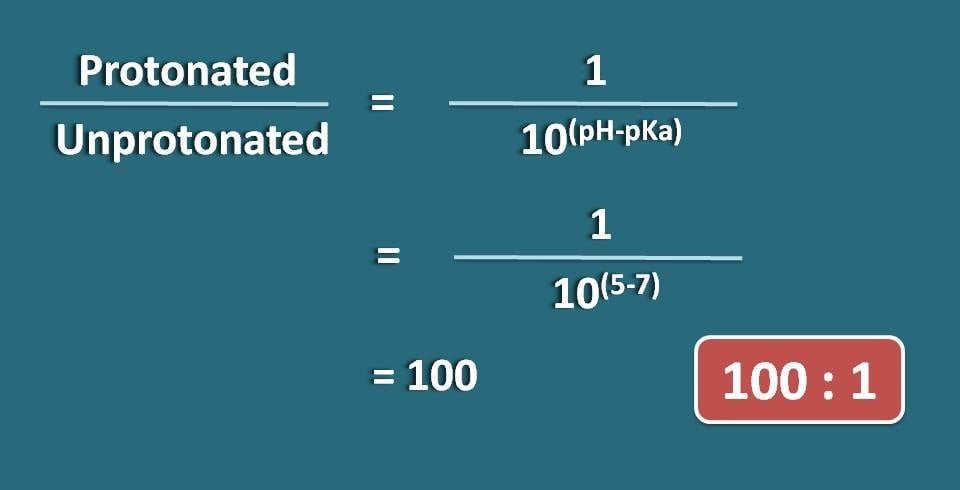So morphine being a basic drug it is mainly exists as protonated form at pH 5 which is relatively acidic pH.